# Converting Improper Fractions To Mixed Numbers Worksheet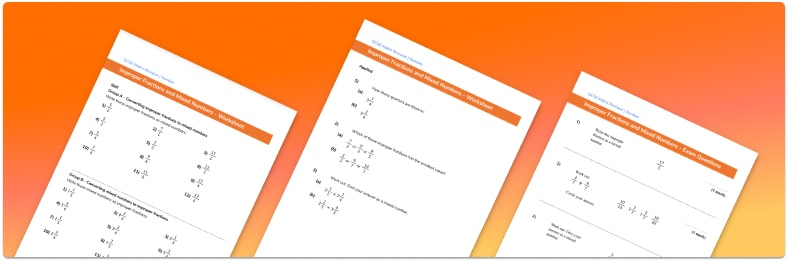• Section 1 of the improper fractions and mixed numbers worksheet contains 36 skills based improper fractions and mixed numbers questions, in 3 groups to support differentiation
• Section 2 contains 3 applied improper fractions and mixed numbers questions with a mix of worded problems and deeper problem solving questions
• Section 3 contains 3 foundation and higher level GCSE exam style improper fractions and mixed numbers questions
• Answers and a mark scheme for all improper fractions and mixed numbers questions
• Follows variation theory with plenty of opportunities for students to work independently at their own level
• All questions created by fully qualified expert secondary maths teachers
• Suitable for GCSE maths revision for AQA, OCR and Edexcel exam boards

• This field is for validation purposes and should be left unchanged.

You can unsubscribe at any time (each email we send will contain an easy way to unsubscribe). To find out more about how we use your data, see our privacy policy.

### Converting improper fractions to mixed numbers at a glance

A proper fraction is one where the numerator is smaller than the denominator. A mixed number (sometimes called a mixed fraction) has both a whole number part and a proper fraction part. An improper fraction is one where the numerator is larger than the denominator ; they are informally known as top-heavy fractions.

In order to carry out fraction arithmetic it is useful to be able to convert a mixed number to an improper fraction, and vice versa. For example, to convert 2 and a fifth into an improper fraction we have to write the whole number 2 in fifths. We know that 5 fifths make a whole, so 10 fifths will make 2 whole numbers. Adding 10 fifths to 1 fifth we get 11 fifths, so 2 and the mixed number 2 and a fifth is equivalent to the improper fraction of 11 fifths.

In order to convert an improper fraction to a mixed number we need to divide the numerator by the denominator. For example, to convert 7 quarters from an improper fraction to a mixed number, we need to divide 7 by 4. 4 goes into 7 once leaving a remainder of 3 quarters. So the improper fraction of 7 quarters is equivalent to the mixed number 1 and 3 quarters.

Looking forward, students can then progress to additional number worksheets, for example aor aFor more teaching and learning support on Number our GCSE maths lessons provide step by step support for all GCSE maths concepts.

## Related worksheets

Converting Mixed Numbers To Improper Fractions Worksheet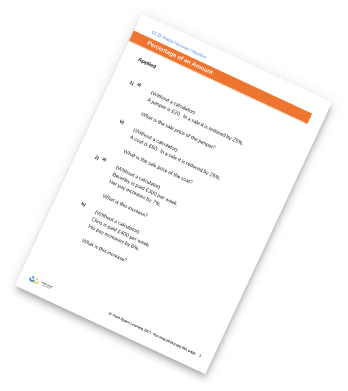Dividing Fractions Worksheet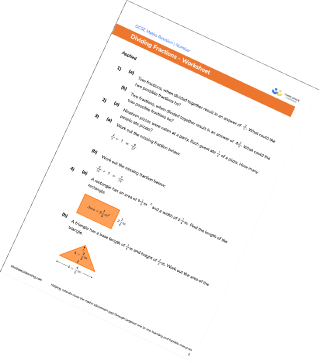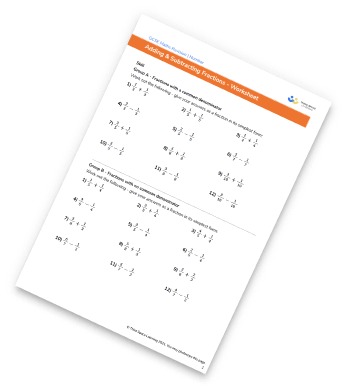Multiplying And Dividing Fractions Worksheets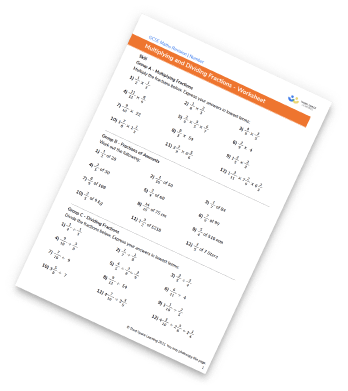## Do you have KS4 students who need more focused attention to succeed at GCSE?There will be students in your class who require individual attention to help them succeed in their maths GCSEs. In a class of 30, it’s not always easy to provide.

Help your students feel confident with exam-style questions and the strategies they’ll need to answer them correctly with our dedicated GCSE maths revision programme.

Lessons are selected to provide support where each student needs it most, and specially-trained GCSE maths tutors adapt the pitch and pace of each lesson. This ensures a personalised revision programme that raises grades and boosts confidence.

Find out more